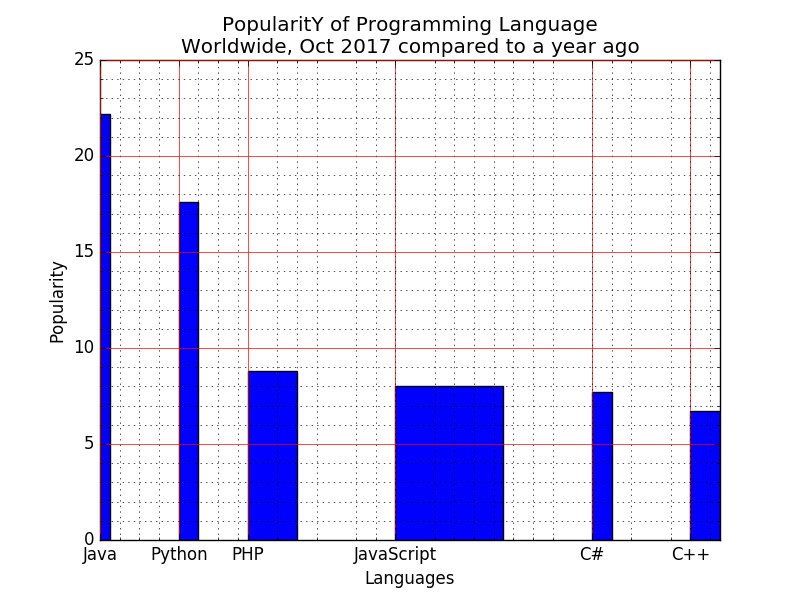﻿ Matplotlib Bar Chart: Display a bar chart of the popularity of programming Languages and select the width of each bar and their positions - w3resource# Matplotlib Bar Chart: Display a bar chart of the popularity of programming Languages and select the width of each bar and their positions

## Matplotlib Bar Chart: Exercise-8 with Solution

Write a Python programming to display a bar chart of the popularity of programming Languages. Select the width of each bar and their positions.

Sample data:
Programming languages: Java, Python, PHP, JavaScript, C#, C++
Popularity: 22.2, 17.6, 8.8, 8, 7.7, 6.7

Sample Solution:

Python Code:

``````import matplotlib.pyplot as plt
x = ['Java', 'Python', 'PHP', 'JavaScript', 'C#', 'C++']
popularity = [22.2, 17.6, 8.8, 8, 7.7, 6.7]
x_pos = [i for i, _ in enumerate(x)]
plt.xlabel("Languages")
plt.ylabel("Popularity")
plt.title("PopularitY of Programming Language\n" + "Worldwide, Oct 2017 compared to a year ago")
plt.xticks(x_pos, x)
# Select the width of each bar and their positions
width = [0.1,0.2,0.5,1.1,0.2,0.3]
y_pos = [0,.8,1.5,3,5,6]

# Create bars
plt.bar(y_pos, popularity, width=width)
plt.xticks(y_pos, x)
# Turn on the grid
plt.minorticks_on()
plt.grid(which='major', linestyle='-', linewidth='0.5', color='red')
# Customize the minor grid
plt.grid(which='minor', linestyle=':', linewidth='0.5', color='black')
plt.show()
```
```

Sample Output:Python Code Editor: